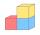# Cube root + expression of a variable from the formula - math problems

#### Number of problems found: 48

• Cube into cylinderIf we dip a wooden cube into a barrel with a 40cm radius, the water will rise 10 cm. What is the size of the cube edge?
• Cube surface and volumeThe surface of the cube is 500 cm2, how much cm3 will be its volume?
• The volume 2The volume of a cube is 27 cubic meters. Find the height of the cube.
• Cube diagonalsCalculate the length of the side and the diagonals of the cube with a volume of 27 cm3.
• Area to volumeIf the surface area of a cube is 486, find its volume.
• Body diagonalCalculate the cube volume, whose body diagonal size is 75 dm. Draw a picture and highlight the body diagonal.
• Cube surface and volumeFind the surface of the cube with a volume of 27 dm3.
• Minimum surfaceFind the length, breadth, and height of the cuboid-shaped box with a minimum surface area, into which 50 cuboid-shaped blocks, each with length, breadth, and height equal to 4 cm, 3 cm, and 2 cm respectively, can be packed.
• Pyramid cutWe cut the regular square pyramid with a parallel plane to the two parts (see figure). The volume of the smaller pyramid is 20% of the volume of the original one. The bottom of the base of the smaller pyramid has a content of 10 cm2. Find the area of the
• CalculateCalculate the surface of the cube if its volume is 64 cm ^ 3.
• CalculateCalculate the length of the wall diagonal of the cube with an edge 5 cm long.
• Cuboid edges in ratioCuboid edges lengths are in ratio 2:4:6. Calculate their lengths if you know that the cuboid volume is 24576 cm3.
• Body diagonalThe cuboid has a volume of 32 cm3. Its side surface area is double as one of the square bases. What is the length of the body diagonal?
• Cube and sphereCube with the surface area 150 cm2 is described sphere. What is sphere surface?
• Area of a cubeCalculate the surface area of a cube if its volume is equal to 729 cubic meters.
• Cube edgeDetermine the edges of the cube when the surface is equal to 37.5 cm square.
• Volume and areaWhat is the volume of a cube which has an area of 361 cm2?
• Prism basesVolume perpendicular quadrilateral prism is 360 cm3. The edges of the base and height of the prism are in the ratio 5:4:2. Determine the area of the base and walls of the prism.
• The cubeThe cube has a surface area of 486 m ^ 2. Calculate its volume.
• Tetrahedral prismThe height of a regular tetrahedral prism is three times greater than the length of the base edge. Calculate the length of the base edge, if you know that the prism volume is 2187 cm3.

Do you have an exciting math question or word problem that you can't solve? Ask a question or post a math problem, and we can try to solve it.

We will send a solution to your e-mail address. Solved examples are also published here. Please enter the e-mail correctly and check whether you don't have a full mailbox.

Cube root - math problems. Expression of a variable from the formula - math problems.